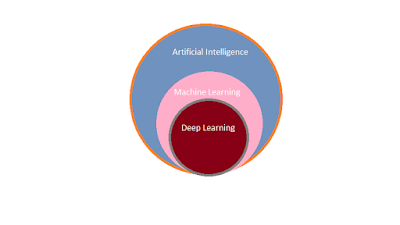Introduction To Artificial Intelligence

Artificial Intelligence is a way of making a computer, a computer-controlled robot, or a software think intelligently, in the similar manner the intelligent humans think.

Applications  of AI:
AI can be implemented in various fields, such as in a self driving car, Cancer Prediction, Robot as Lecturer  etc.

Two Subsets of AI:Artificial Intelligence

1. Machine Learning
2. Deep Learning

1. Machine Learning  :

Machine Learning is a subset of Artificial Intelligence.
ML systems learn how to combine input to produce useful predictions on never-before-seen data.
In Machine Learning , We train a model using a large set of labeled data, later on this model is used to test a set of test data i.e unlabeled data. Then accuracy is calculated that how accurate  the prediction is.

Suppose that we need an AI to check whether this object is dog or not, then we provide various sets of  sample inputs during the training of our model.
inputs consist of feature and label
Dog can be identified by the way they: speak, run, height, eyes, tail etc.
Here label is DOG and Features are sound, speed, height, and eye.

When we say labeled data it means that a large data set  that contains Feature and Label.
So ML moves around mainly three terminologies:
(i) Label  (ii) Feature (iii) Model

(i) Label:

A label is the thing we're predicting—the y variable in simple linear regression. The label could be the future price of wheat, the kind of animal shown in a picture, the meaning of an audio clip, or just about anything.

(ii) Feature:

A feature is an input variable—the x variable in simple linear regression. A simple machine learning project might use a single feature, while a more sophisticated machine learning project could use millions of features, specified as:  x1, x2, ...., xn
Feature could be a property possessed by that thing which is useful to identify that thing uniquely/partially.

(iii) Model:

A model defines the relationship between features and label.

• Training means creating or learning the model. That is, you show the model labeled examples and enable the model to gradually learn the relationships

between features and label.
• Inference means applying the trained model to unlabeled examples. That is, you use the trained model to make useful predictions (y').

Labeled Example:

This example is an instance of training data.
{x, y} or      {Feature, Label}

In order to train our model, we fed a large set of such instances with features and labels.

Unlabeled Example:

This example is an instance of testing data.
{x, ?}  or   {Feature, ?}
Finally we get a trained model that takes the unlabeled test data and make predictions for each test data.

"Regression vs. classification"

A regression model predicts continuous values. For example, regression models make predictions that answer questions like the following:
• What is the value of a house in California?
• What is the probability that a user will click on this ad?

A classification model predicts discrete values. For example, classification models make    predictions that answer questions like the following:
• Is a given email message spam or not spam?
• Is this an image of a dog, a cat, or a hamster?

Model can learn using two approaches: Supervised Learning and Unsupervised Learning.

" In supervised learning model is being trained under the supervision of input labeled data.But in unsupervised learning no hint is provided during the training of model. Model learns by its own."

"Machine Learning changes the way you think about a problem. The focus shifts from a mathematical science to a natural science, running experiments and using statistics, not logic, to analyse its results." - Peter Norvig - Google Research Director